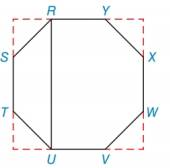Chapter 7.3, Problem 29E### Elementary Geometry for College St...

6th Edition
Daniel C. Alexander + 1 other
ISBN: 9781285195698

#### Solutions

Chapter
Section### Elementary Geometry for College St...

6th Edition
Daniel C. Alexander + 1 other
ISBN: 9781285195698
Textbook Problem
1 views

# Given regular octagon RSTUVWXY with each side of length 4, find the length of diagonal RU. (HINT: Extended sides, as shown, form a square.)To determine

To find:

The length of the diagonal RU.

Explanation

Consider the given figure.

Here, ABCD is a square with all vertex angles equal to 180°.

All four triangles are isosceles right triangle.

In triangle ASR, AS = AR

Use Pythagoras theorem in triangle ASR.

AR2+AS2=RS2AS2+AS2=422AS2=16AS2=8AS=22

In triangle DTU, DT = DU

Use Pythagoras theorem in triangle DTU.

DT2+DU2=TU2DT2+D

### Still sussing out bartleby?

Check out a sample textbook solution.

See a sample solution

#### The Solution to Your Study Problems

Bartleby provides explanations to thousands of textbook problems written by our experts, many with advanced degrees!

Get Started

#### Differentiate the function. f(x) = x ln x x

Single Variable Calculus: Early Transcendentals, Volume I

#### In Exercises 2124, factor each expression completely. 23. 16 x2

Applied Calculus for the Managerial, Life, and Social Sciences: A Brief Approach

#### True or False: If h'(x) = k(x), then k(x) is an antiderivative of h(x).

Study Guide for Stewart's Single Variable Calculus: Early Transcendentals, 8th

#### The gradient vector field for is:

Study Guide for Stewart's Multivariable Calculus, 8th

#### Graph each equation. 4+3y=3(x+y)

College Algebra (MindTap Course List)